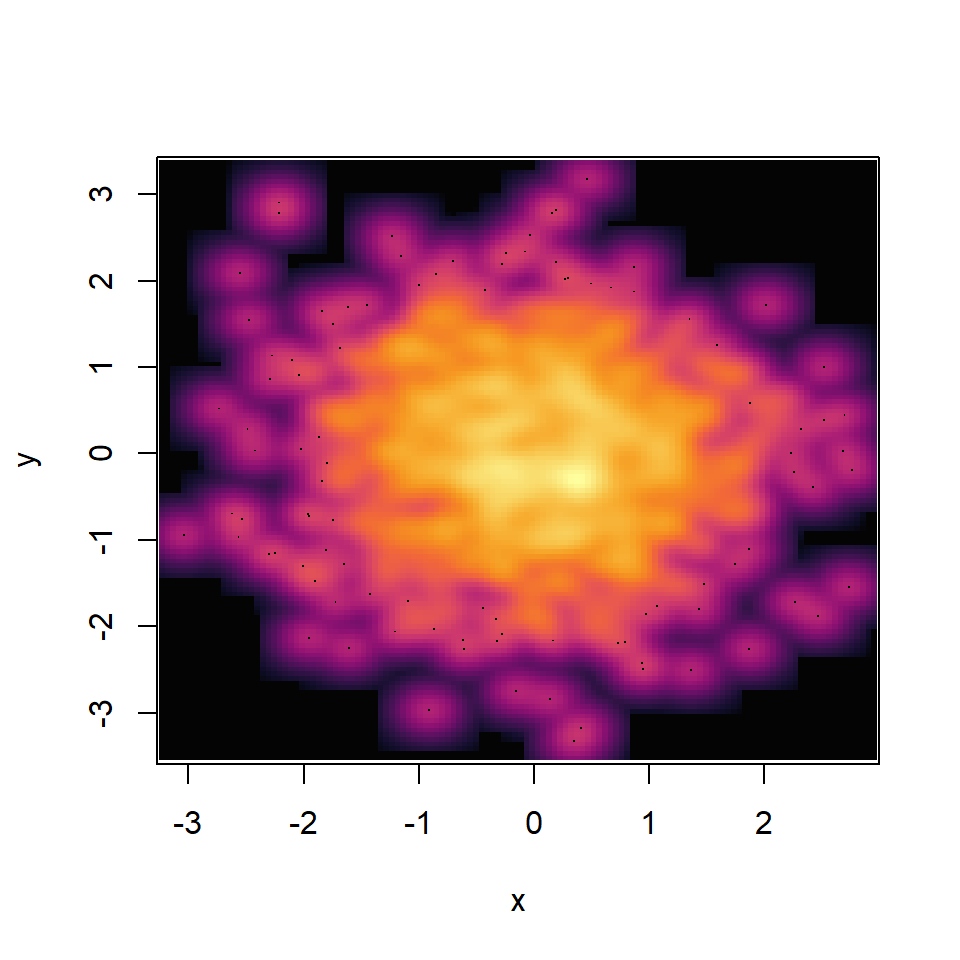# Smooth scatter plot in R

## `smoothScatter` function

Creating a smooth scatter plot in base R is straightforward with `smoothScatter` function. You just need to pass your vectors to the function and a smoothed version of its scatter plot will be created.

``````# Data
set.seed(9)
x <- rnorm(1000)
y <- rnorm(1000)

# Smooth scatter plot
smoothScatter(y ~ x)

# Equivalent to:
smoothScatter(x, y)``````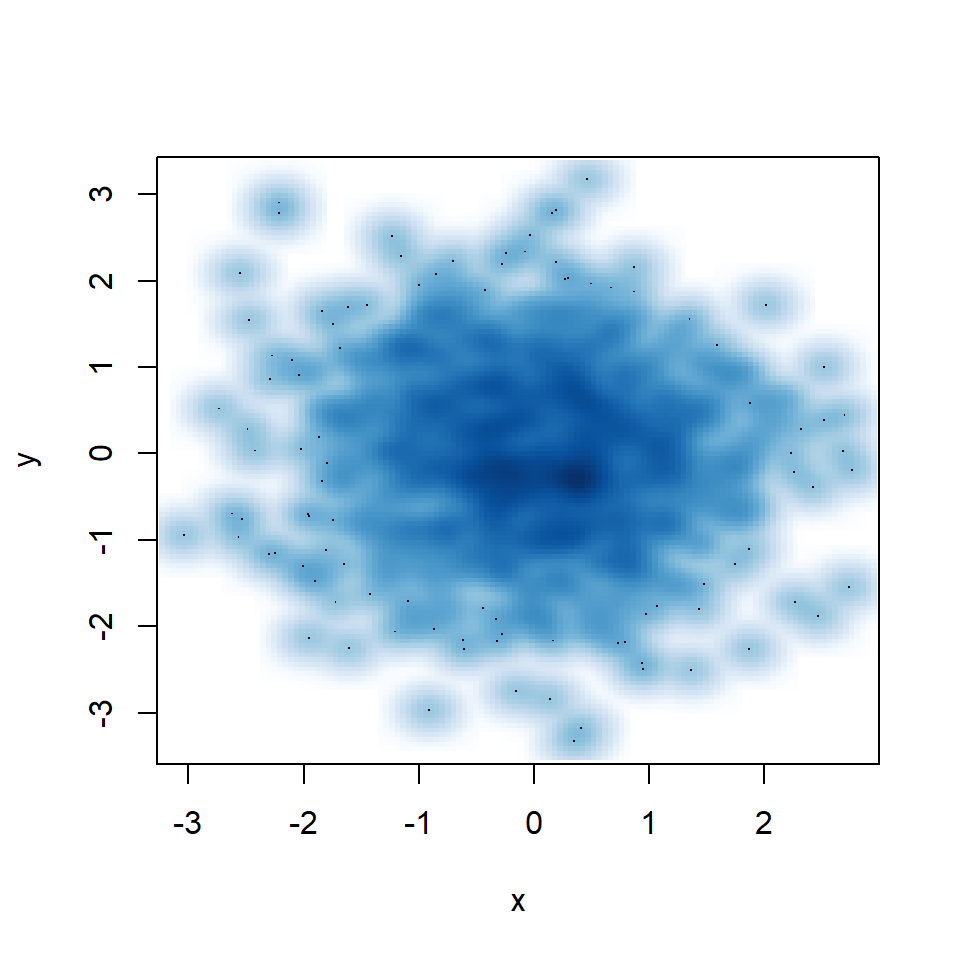You might have noticed that some points are displayed on the graph, which are points from those areas of lowest regional densities. These points are useful for outlier identification. The number of points can be customized with `nrpoints`.

``````# Data
set.seed(9)
x <- rnorm(1000)
y <- rnorm(1000)

# Smooth scatter plot
smoothScatter(y ~ x,
nrpoints = 1000)``````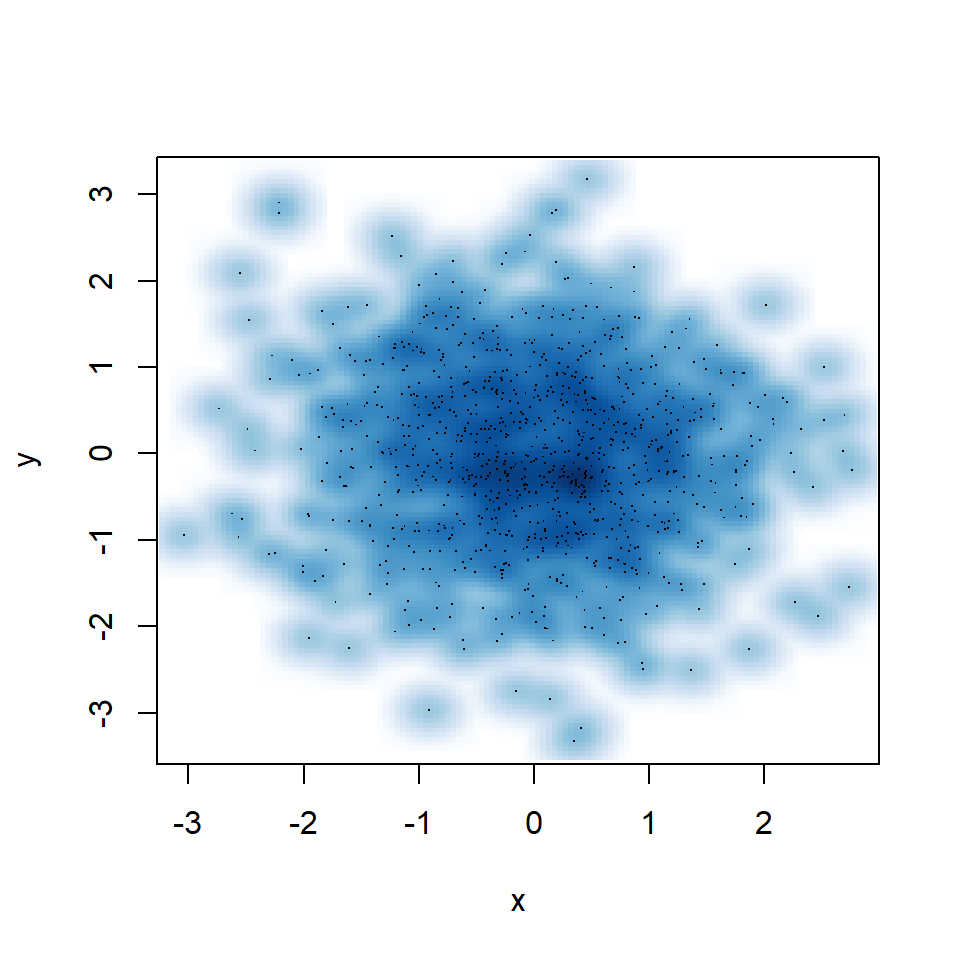In addition, the pch symbol and its color can be customized with `pch` and `col` arguments.

``````# Data
set.seed(9)
x <- rnorm(1000)
y <- rnorm(1000)

# Smooth scatter plot
smoothScatter(y ~ x,
pch = 10, col = "red")``````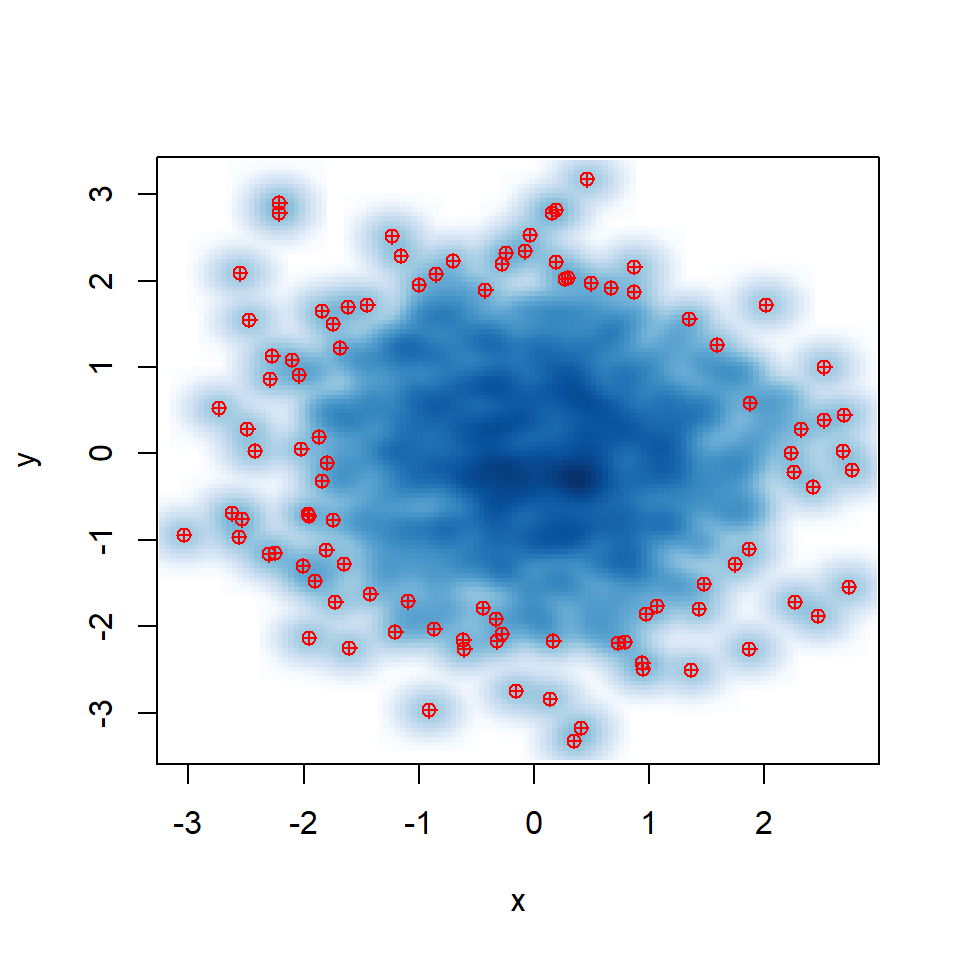### Smooth scatter bandwidth

The `smoothScatter` function depends on `bkde2D` function from package `KernSmooth` to perform the two-dimensional kernel density estimate. However, you can also set a custom smoothing bandwidth with `bandwidth` argument.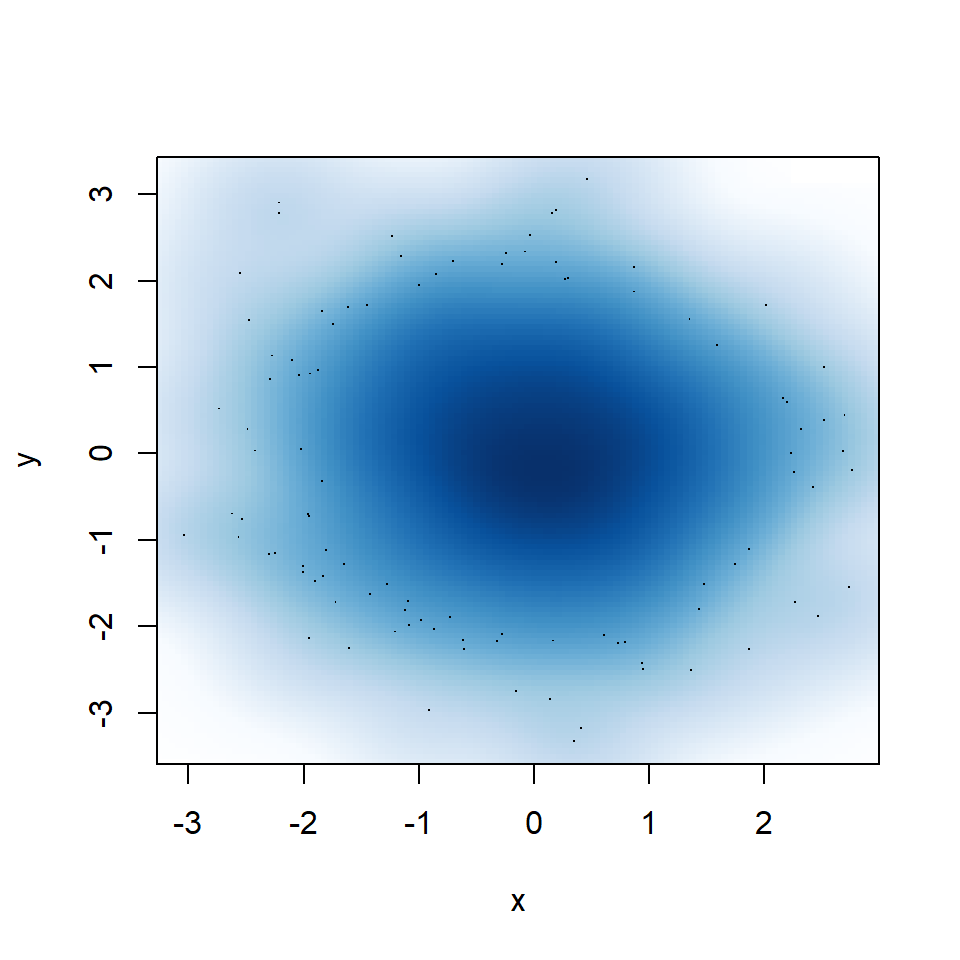Big bandwidth

If you select a too big bandwidth the estimation will be oversmoothed.

``````# Data
set.seed(9)
x <- rnorm(1000)
y <- rnorm(1000)

# Smooth scatter plot
smoothScatter(y ~ x,
bandwidth = 0.4) # Big bandwidth``````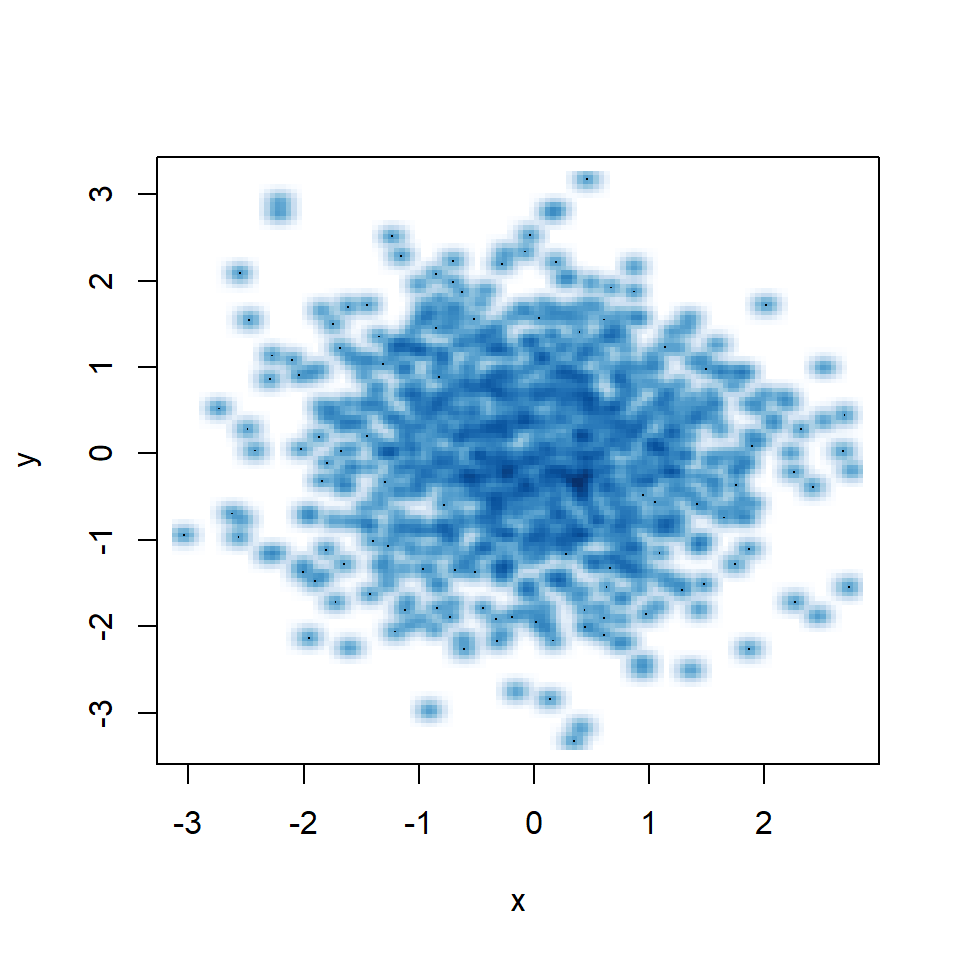Small bandwidth

A small bandwidth will group the data too much, so the kernel density estimation won’t be smooth.

``````# Data
set.seed(9)
x <- rnorm(1000)
y <- rnorm(1000)

# Smooth scatter plot
smoothScatter(y ~ x,
bandwidth = 0.05) # Small bandwidth``````

### Smooth scatter color

The function uses the `blues9` color palette, but you can choose your own specifying a color ramp palette as in the following example. See our color palettes list for inspiration.

``````# Data
set.seed(9)
x <- rnorm(1000)
y <- rnorm(1000)

palette <- hcl.colors(30, palette = "inferno")

# Smooth scatter plot
smoothScatter(y ~ x,
colramp = colorRampPalette(palette))``````